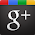Infix Expression :

Any expression in the standard form like "2*3-4/5" is an Infix(Inorder) expression.

Postfix Expression :

The Postfix(Postorder) form of the above expression is "23*45/-".

Example :

• infix (1+2)*(3+4)
• with parentheses: ((1+2)*(3+4))
• in postfix: 12+34+*
• in prefix: *+12+34
• infix 1^2*3-4+5/6/(7+8)
• paren.: ((((1^2)*3)-4)+((5/6)/(7+8)))
• in postfix: 12^3*4-56/78+/+
• in prefix: +-*^1234//56+78
• Scan the Infix string from left to right.
• Initialise an empty stack.
• If the scannned character is an operand, add it to the Postfix string. If the scanned character is an operator and if the stack is empty Push the character tostack.
• If the scanned character is an Operand and the stack is not empty, compare the precedence of the character with the element on top of the stack(topStack). If topStack has higher precedence over the scanned character Popthe stack else Push the scanned character to stack. Repeat this step as long as stack is not empty and topStack has precedence over the character.
• (After all characters are scanned, we have to add any character that the stackmay have to the Postfix string.) If stack is not empty add topStack to Postfix stringand Pop the stack. Repeat this step as long as stack is not empty.
• Return the Postfix string.

Infix to Postfix Conversion :

In normal algebra we use the infix notation like a+b*c. The corresponding postfix notation is abc*+.
The algorithm for the conversion is as follows :
Repeat this step till all the characters are scanned.

Java version of infix to postfix  is given bellow :

/**
* To change this template, choose Tools | Templates and open the template in
* the editor.
*/
package stack;

import java.util.Scanner;

/**
*
* @author ACHCHUTHAN
*/
public class Infix2Postfix extends Stack {

public Infix2Postfix(int x) {
super(x);
}

/**
*@return postfixString value
*/

public String InToPost(String infixString) {
String postfixString = " ";

for (int index = 0; index < infixString.length(); ++index) {
char chValue = infixString.charAt(index);
if (chValue == '(') {
push('(');
} else if (chValue == ')') {
Character oper = peek();
while (!(oper.equals('(')) && !(isEmpty())) {
postfixString += oper.charValue();
pop();
oper = peek();
}
pop();
} else if (chValue == '+' || chValue == '-') {
//Stack is empty
if (isEmpty()) {
push(chValue);
//current Stack is not empty
} else {
Character oper = peek();
while (!(isEmpty() || oper.equals(new Character('(')) || oper.equals(new Character(')')))) {
pop();
postfixString += oper.charValue();
}
push(chValue);
}
} else if (chValue == '*' || chValue == '/') {
if (isEmpty()) {
push(chValue);
} else {
Character oper = peek();
while (!oper.equals(new Character('+')) && !oper.equals(new Character('-')) && !isEmpty()) {
pop();
postfixString += oper.charValue();
}
push(chValue);
}
} else {
postfixString += chValue;
}
}
while (!isEmpty()) {
Character oper = peek();
if (!oper.equals(new Character('('))) {
pop();
postfixString += oper.charValue();
}
}
return postfixString;
}

public static void main(String[] args) {
Infix2Postfix mystack = new Infix2Postfix(10);
System.out.println("Type in an expression like (1+2)*(3+4)/(12-5)\n "
+ "with no monadic operators like in-5 or +5 followed by key");
Scanner scan = new Scanner(System.in);
String str = scan.next();
System.out.println("The Expression you have typed in infix form :\n"+str);
System.out.println("The Equivalent Postfix Expression is :\n"+mystack.InToPost(str));
}
}
Output of this program :

run:
Type in an expression like (1+2)*(3+4)/(12-5)
with no monadic operators like in-5 or +5 followed by <ENTER>key
(1+2)*(3+4)
The Expression you have typed in infix form :
(1+2)*(3+4)
The Equivalent Postfix Expression is :
12+34+*
BUILD SUCCESSFUL (total time: 28 seconds)

1.i have execute this program. but i am getting one error
that is
---------- javac ----------
Infix2Postfix.java:4: cannot find symbol
symbol: class character
public class Infix2Postfix extends Stack {
^
1 error

Output completed (0 sec consumed) - Normal Termination

2.in this program you need to extends Stack;better you create stack general type class and extends that one to this program .good luck

http://java90.blogspot.com/2012/01/stack-data-structure-in-java.html

Thank you for vising Java90.blogspot.com